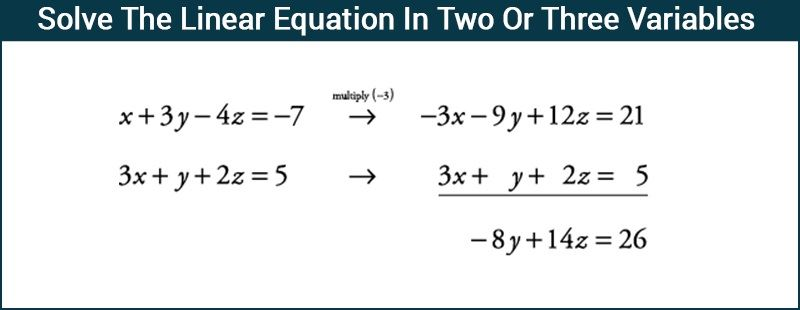# Solve The Linear Equation In Two Or Three Variables

A linear equation in two variables is of the form ax + by + c = 0, where a, b, and c are constants (real numbers). Similarly, a linear equation in three variables is of the form ax + by + cz + d = 0, where a, b, c, and d are constants. We have different methods of solving given linear equations in two or three variables in maths. Let us first understand why we prefer using matrices to solve a system of linear equations?

Consider the linear equation in one variable 5x = 2x + 3. We can easily figure out x = 1. When we are given a pair of linear equations consisting of two variables, we use the simultaneous linear equations concept to find out the value of the unknown variables. Similarly, suppose we have a number of linear equations consisting of a number of variables. In that case, finding out the value of the unknown variables becomes tedious and complex. To solve such equations, the use of an inverse matrix is comparatively easier.

There are three methods of solving linear equations in two variables. They are:

Now you will learn how to use an inverse matrix to solve the system of linear equations in three variables.## Solving Linear Equations Using Inverse Matrix

Consider the system of equations:

$$\begin{array}{l} a_1 x + b_1 y + c_1 z = d_1 \end{array}$$

$$\begin{array}{l} a_2 x + b_2 y + c_2 z = d_2 \end{array}$$
,

$$\begin{array}{l} a_3 x + b_3 y + c_3 z = d_3 \end{array}$$

where

$$\begin{array}{l} d_1, d_2, d_3\end{array}$$
are not all zero.

Let

$$\begin{array}{l} A = \begin{bmatrix} a_1 & b_1 & c_1\cr a_2 & b_2 & c_2\cr a_3 & b_3 & c_3 \end{bmatrix}\end{array}$$

$$\begin{array}{l}B = \begin{bmatrix} x\cr y\cr z \end{bmatrix}\end{array}$$

$$\begin{array}{l}C = \begin{bmatrix} d_1\cr d_2\cr d_3 \end{bmatrix}\end{array}$$

A is known as the coefficient matrix of the system of linear equations.

We can write the given equations in as follows:

$$\begin{array}{l}\begin{bmatrix} a_1 & b_1 & c_1\cr a_2 & b_2 & c_2\cr a_3 & b_3 & c_3 \end{bmatrix}\begin{bmatrix} x\cr y\cr z \end{bmatrix} \begin{bmatrix} d_1\cr d_2\cr d_3 \end{bmatrix}\end{array}$$

We can abbreviate the above system of equations as, AX = B , i.e.

Now two cases arise which are explained further,

Case I: If A  is non-singular matrix

We know that if a matrix is non-singular (i.e. the value of determinant of the matrix is not zero) then the inverse of such a matrix exists.

Since AX = B

Multiplying both sides by A-1

$$\begin{array}{l}\Rightarrow\end{array}$$
A-1(AX) = A -1B

$$\begin{array}{l}\Rightarrow\end{array}$$
(A-1A)X = A -1B

(Using associative property)

$$\begin{array}{l}\Rightarrow\end{array}$$
X = A-1B

As inverse of a matrix is unique therefore this matrix equation gives unique solution for the given system of linear equations.

Case II: If  A is a singular matrix

If a matrix is singular, then the determinant of A  is zero. Then we will have to calculate the value of

$$\begin{array}{l} (adj A)B \end{array}$$
.

Now, in a case when

$$\begin{array}{l} (adj A)B \neq 0\end{array}$$
, then the solution does not exists and the system of equations is known as inconsistent.

On the other hand, if

$$\begin{array}{l}(adj A)B = 0\end{array}$$
, then the given system of linear equations could either be inconsistent or consistent system. Also, the system could have either infinitely many solutions or no solution. Let us see an example to understand this concept better.

### Example

Example:

Solve the given system of linear equation

$$\begin{array}{l}x + 3y – 2z = 10\end{array}$$

$$\begin{array}{l} 2x – y + 6z = 3\end{array}$$

$$\begin{array}{l} x + y – 2z = 5\end{array}$$

Solution:

We can write the above equations in form of matrix as follows:

$$\begin{array}{l}AX = B\end{array}$$

$$\begin{array}{l}\begin{bmatrix} 1 & 3 & -2\cr 2 & -1 & 6\cr 1 & 1 & -2 \end{bmatrix}\begin{bmatrix} x\cr y\cr z \end{bmatrix} = \begin{bmatrix} 10\cr 3\cr 5 \end{bmatrix}\end{array}$$

To find the matrix

$$\begin{array}{l}X\end{array}$$
we need to find the inverse of matrix  such that
X = A-1 B

Determinant of the matrix A is:

|A| = 1(2 – 6) – 3(-4 – 6) – 2(2 + 1)

= -4 -3(-10) -2(3)

= -4 + 30 – 6

= 20

Let us find the adjoint of matrix A.

$$\begin{array}{l}Adj (A)=\begin{bmatrix} -4 & 4 &16 \\ 10 &0 &-10 \\ 3& 2 &-7 \end{bmatrix}\end{array}$$

A-1 =

$$\begin{array}{l}\begin{bmatrix} \frac{-1}{5} & \frac{1}{5} & \frac{4}{5}\cr \frac{1}{2} & 0 & \frac{-1}{2}\cr \frac{3}{20} & \frac{1}{10} & \frac{-7}{20} \end{bmatrix}\end{array}$$

$$\begin{array}{l}\Rightarrow \end{array}$$
X = A-1B =
$$\begin{array}{l} \begin{bmatrix} \frac{-1}{5} & \frac{1}{5} & \frac{4}{5}\cr \frac{1}{2} & 0 & \frac{-1}{2}\cr \frac{3}{20} & \frac{1}{10} & \frac{-7}{20} \end{bmatrix}\begin{bmatrix} 10\cr 3\cr 5 \end{bmatrix}\end{array}$$

$$\begin{array}{l}\Rightarrow\end{array}$$
X =
$$\begin{array}{l}\begin{bmatrix} x\cr y\cr z \end{bmatrix} \end{array}$$
=
$$\begin{array}{l}\begin{bmatrix} \frac{60}{23}\cr \frac{57}{23}\cr \frac{1}{23} \end{bmatrix}\end{array}$$

Thus the solution of the above equations is:

$$\begin{array}{l}x = \frac{60}{23}\end{array}$$

$$\begin{array}{l}y = \frac{57}{23}\end{array}$$

$$\begin{array}{l}z = \frac{1}{23}\end{array}$$

### Practice Problems

Solve the following questions to get a thorough understanding of the method of solving linear equations in three variables using matrix inverse.

1. Solve the following system of linear equations in three variables.
-3x + 2y – 5z = -14
2x – 3y + 4z = 10
x + y + z = 4
2. Find the solution of the system of three linear equations in three variables.
3x – 4y + 2z = -9
-4x + 4y + 10z = 32
-x + 2y – 7z = -7
3. What is the solution of the following system of linear equations?
4a + 3b = 4
2a + 2b – 2c = 0
5a + 3b + c = -2

Let us learn hand in hand with BYJU’s and explore the depths of knowledge in a way you have never thought before. Please download our app-BYJU’s-The Learning App to keep learning.